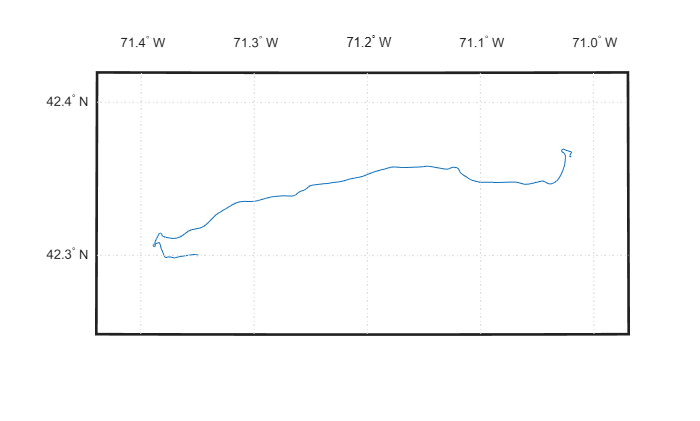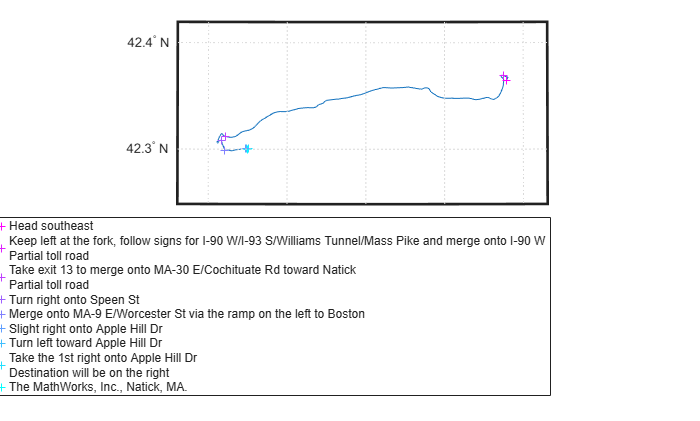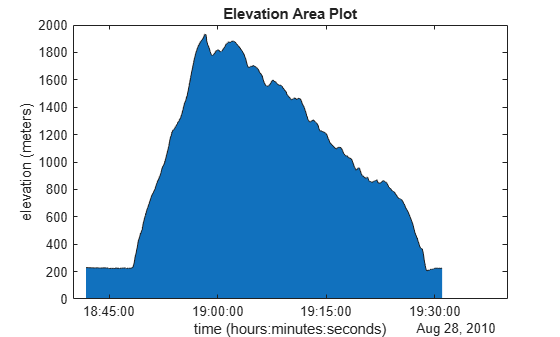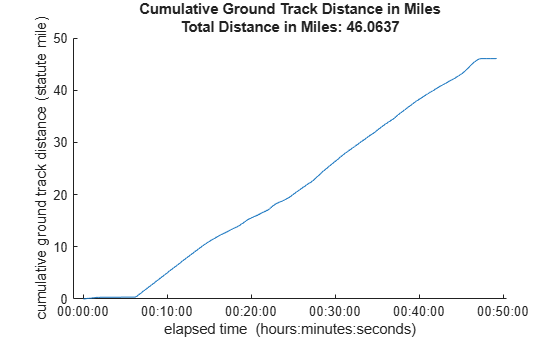## Syntax

``P = gpxread(filename)``
``P = gpxread(URL)``
``S = gpxread(___,'Index',V)``
``___ = gpxread(___,Name,Value)``

## Description

example

````P = gpxread(filename)` reads point data from the GPS Exchange Format (GPX) file, `filename`, and returns an n-by-1 geopoint vector, `P`, where n is the number of waypoints, or points that define a route or track. `gpxread` searches the file first for waypoints, then routes, and then tracks, and it returns the first type of data it finds. The Metadata field of `P` identifies the feature type (`'waypoint'`, `'track'`, or `'route'`) and any additional metadata associated with waypoint, route, or track. If the file contains multiple tracks or routes, `P` contains the points that define the first track or route in the file. If `gpxread` cannot find any features in the file, it returns an empty geopoint vector.```
````P = gpxread(URL)` reads the GPX data from a `URL`. The `URL` must include the protocol type (for example, `http://`).```

example

````S = gpxread(___,'Index',V)` returns data from the GPX file in a geoshape vector, rather than a geopoint vector, only if the file contains track or route data and you specify the value of `'Index'` as a vector, `V`. Use this syntax when you want to work with the data as a line, rather than as a collection of points.```

example

````___ = gpxread(___,Name,Value)` reads data from a GPX file with additional options, specified by one or more `Name,Value` pair arguments, that control various characteristics of the import. `Name` is the argument name and `Value` is the corresponding value. `Name` must appear inside single quotes `('')` and is case insensitive. You can specify several name-value pair arguments in any order.```

## Examples

collapse all

Read and display waypoints from the `boston_placenames.gpx` file and overlay the points onto the `boston.tif` image.

First, import the waypoints and GeoTIFF file.

```p = gpxread('boston_placenames.gpx'); [A,R] = readgeoraster('boston.tif');```

Get the projection structure of the GeoTIFF image. Convert the length unit of the X and Y limits to meters for use with the projection structure.

```proj = geotiffinfo('boston.tif'); mstruct = geotiff2mstruct(proj); R.XWorldLimits = R.XWorldLimits * proj.UOMLengthInMeters; R.YWorldLimits = R.YWorldLimits * proj.UOMLengthInMeters;```

Display the map.

```axesm(mstruct) mapshow(A,R)```Display the names and positions of each point.

```for k=1:length(p) textm(p(k).Latitude, p(k).Longitude, p(k).Name, ... 'Color','k','BackgroundColor',[0.9 0.9 0],... 'Interpreter','none'); end geoshow(p) xlim(R.XWorldLimits) ylim(R.YWorldLimits)```Read and display a route from Boston Logan International Airport to MathWorks in Natick, MA.

Read the route information from the GPX file.

`route = gpxread('sample_route.gpx');`

Compute `latlim` and `lonlim` with a 0.05 buffer.

```[latlim, lonlim] = geoquadline(route.Latitude, route.Longitude); [latlim, lonlim] = bufgeoquad(latlim, lonlim, .05, .05);```

Display the route.

```fig = figure; pos = fig.Position; fig.Position = [300 300 1.25*pos(3) 1.25*pos(4)]; ax = usamap(latlim, lonlim); setm(ax, 'MLabelParallel', 43.5) geoshow(route.Latitude, route.Longitude)```Extract the elements of route that include descriptions of turns, mark and color code each turn on the map, and construct a legend that displays the descriptions. Reverse the order, so that the legend displays the first turn at the top and the last at the bottom.

```turns = route(~cellfun(@isempty, route.Description)); turns = turns(end:-1:1); n = length(turns); colors = cool(n); for k=1:n geoshow(turns(k).Latitude, turns(k).Longitude, ... 'DisplayType','point','MarkerEdgeColor',colors(k,:),... 'Tag','turn','DisplayName',turns(k).Description) end legend(findobj(ax,'Tag','turn'),'Location','SouthOutside')```Read track log from a GPX file and display overlaid on a web map.

Read the track logs from a GPX file. `gpxread` returns the data in a geoshape object.

```tracks = gpxread('sample_tracks', 'Index', 1:2); ```

Display the track logs on a web map with a different color for each track log.

```webmap('openstreetmap') colors = {'cyan', 'red'}; wmline(tracks, 'Color', colors) ```Zoom the web map to view the first track near the MathWorks campus in Natick.

```[latlim, lonlim] = geoquadline(tracks(1).Latitude, tracks(1).Longitude); wmlimits(latlim, lonlim) ```Read waypoints and track log from the `sample_mixed.gpx` file.

```wpt = gpxread('sample_mixed'); trk = gpxread('sample_mixed', 'FeatureType', 'track'); ```

Display the waypoints and the track log on a web map.

```webmap('worldimagery') wmline(trk, 'OverlayName', 'Track Logs'); ```Add web markers to mark the positions of each way point.

`wmmarker(wpt, 'FeatureName', wpt.Name, 'OverlayName', 'Waypoints')`This example shows how to display elevation and time area maps and calculate distance using track logs.

Read the track log from the `sample_mixed.gpx` file.

`trk = gpxread('sample_mixed.gpx','FeatureType','track');`

Simplify the format of the time value strings by removing the fractional seconds and time zone offset. Convert the strings to a `datetime` array.

```timeStr = strrep(trk.Time,'.000Z',''); t = datetime(timeStr);```

Display an area plot of the time and elevation values.

```figure area(t,trk.Elevation) xtickformat('HH:mm:ss') xlabel('time (hours:minutes:seconds)') ylabel('elevation (meters)') title('Elevation Area Plot');```Calculate and display ground track distance. Convert distance in meters to distance in U.S. survey miles.

```e = wgs84Ellipsoid; lat = trk.Latitude; lon = trk.Longitude; d = distance(lat(1:end-1), lon(1:end-1), lat(2:end), lon(2:end), e); d = d * unitsratio('sm', 'meter');```

Display the cumulative ground track distance and elapsed time.

```elapsedTime = t - t(1); figure line(elapsedTime(2:end),cumsum(d)) ylabel('cumulative ground track distance (statute mile)') xlabel('elapsed time (hours:minutes:seconds)') title({'Cumulative Ground Track Distance in Miles', ... ['Total Distance in Miles: ' num2str(sum(d))]});```## Input Arguments

collapse all

Name of GPX file to open, specified as a string scalar or character vector. If the file is not in the current folder or in a folder on the MATLAB® path, you must specify the folder path. If the file name includes the extension `'.gpx'` (either uppercase or lowercase), you can omit the extension from `filename`.

Example: `'boston_placenames'`

Data Types: `char` | `string`

Internet location containing GPX data, specified as a URL. The URL must include protocol type (for example, `https://`).

Data Types: `char` | `string`

### Name-Value Arguments

Specify optional comma-separated pairs of `Name,Value` arguments. `Name` is the argument name and `Value` is the corresponding value. `Name` must appear inside quotes. You can specify several name and value pair arguments in any order as `Name1,Value1,...,NameN,ValueN`.

Example: `'FeatureType','track'`

Type of feature to read from file, specified as one of the following: `'track'`, `'route'`, `'waypoint'`, or `'auto'`. If `gpxread` cannot find the specified feature in the file, it returns an empty geopoint vector.

Example: `'FeatureType','waypoint'`

Data Types: `char` | `string`

Index of waypoint, track, or route data in file, specified as a scalar or vector of positive integers.

• If the value is a scalar, `gpxread` returns the specified waypoint, route, or track as a geopoint vector. If the scalar value is greater than the total number of elements found in the file, `gpxread` returns an empty geopoint vector.

• If the value is a vector, and the file contains waypoints, `gpxread` returns those waypoints specified by the vector. If the file contains routes or tracks (and does not contain waypoints), `gpxread` returns the specified routes or track logs in a geoshape vector. `gpxread` sets the Geometry field of the geoshape vector to `'line'`.

Example: `'Index', [1:2]` would read up to two routes or tracks, if the file contained routes or tracks, in a geoshape vector.

Data Types: `single` | `double` | `int8` | `int16` | `int32` | `int64` | `uint8` | `uint16` | `uint32` | `uint64`

## Output Arguments

collapse all

Waypoint, track, or route data, returned as an n-by-1 geopoint vector, where n is the number of points.

For a track log or route with multiple segments, `gpxread` concatenates the coordinates of the segments with `NaN` separators. `NaN` denotes numeric elements not found in the file. The empty character vector `('')` is used to denote text elements not found in the file.

Track or route data, returned as an n-by-1 geopoint vector

collapse all

### waypoint

A point of interest, or named feature on a map.

### track

An ordered list of waypoints that describe a path.

### route

An ordered list of waypoints representing a series of turn points leading to a destination.

## Tips

• Excluding extensions, GPX version 1.1 is fully supported. If any other version is detected, a warning is issued. However, in most cases, version 1.0 GPX files can be read successfully unless they contain certain metadata tags. For more information, see the GPX 1.1 Schema Documentation.